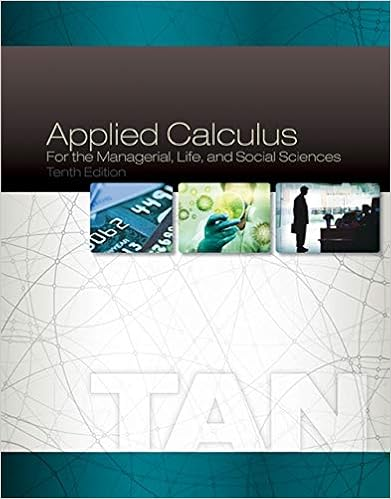# Explain the paradox of thrift with the use of a graph

• Test Prep
• 6
• 100% (13) 13 out of 13 people found this document helpful

This preview shows page 3 - 5 out of 6 pages.

##### We have textbook solutions for you!
The document you are viewing contains questions related to this textbook.The document you are viewing contains questions related to this textbook.
Chapter 6 / Exercise 2
Applied Calculus for the Managerial, Life, and Social Sciences
TanExpert Verified
8. Explain the "paradox of thrift" with the use of a graph assuming that investment is autonomous of aggregate output.
9. If the government wants to reduce unemployment what are some ways it should change spending and taxes?
10. Assume the following behavioral equations for a macroeconomy: C = 100 + .9Y d , I = 50, T = 100 and G = 40 Calculate the equilibrium level of output.
##### We have textbook solutions for you!
The document you are viewing contains questions related to this textbook.The document you are viewing contains questions related to this textbook.
Chapter 6 / Exercise 2
Applied Calculus for the Managerial, Life, and Social Sciences
TanExpert Verified
Rewriting the consumption function yields: 100 + .9 (Y - 100) since Y d = Y - T. This reduces to 10 + .9Y. When added to I and G yields 10 + .9Y + 50 + 40. Combining terms this equals 100 + .9Y. Since this is AE we must then set it equal to Y and solve for Y. We then get 100 + .9Y = Y. Rearranging and solving for Y yields 1000. 11. Determine the impact of an increase in government spending of \$10 billion when the MPC is .9. Determine the impact of a decrease in government spending of \$5 billion when the MPS is .5.
•••